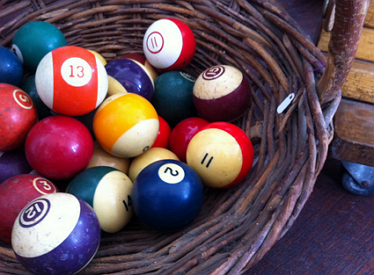We have a number of Balls
if we divide Balls equally on 2 Baskets, there will be 1 Ball left.
if we divide Balls equally on 3 Baskets,there will be 1 Ball left.
if we divide Balls equally on 4 Baskets, there will be 1 Ball left.
if we divide Balls equally on 5 Baskets, there will be 1 Ball left.
if we divide Balls equally on 6 Baskets, there will be 1 ball left.
if we divide Balls equally on 7 Baskets, there will be no balls left.
What is the least possible number of Balls?

×